# MATH PRESENTATON GOLDEN RATO GOLDEN RATIO In math

• Slides: 10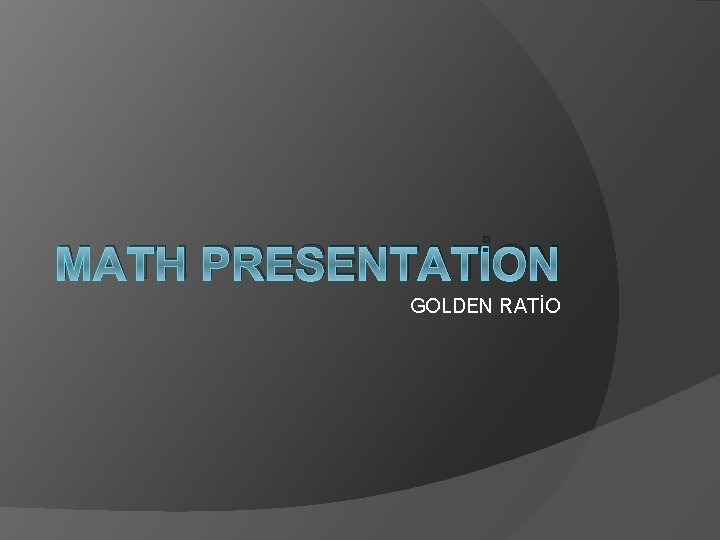MATH PRESENTATİON GOLDEN RATİO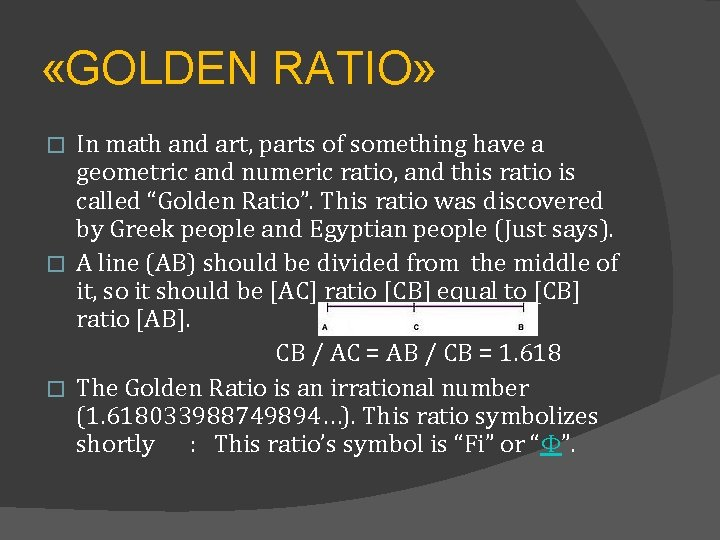«GOLDEN RATIO» In math and art, parts of something have a geometric and numeric ratio, and this ratio is called “Golden Ratio”. This ratio was discovered by Greek people and Egyptian people (Just says). � A line (AB) should be divided from the middle of it, so it should be [AC] ratio [CB] equal to [CB] ratio [AB]. CB / AC = AB / CB = 1. 618 � The Golden Ratio is an irrational number (1. 618033988749894…). This ratio symbolizes shortly : This ratio’s symbol is “Fi” or “Φ”. �GOLDEN RATIO ON HUMAN Golden Ratio on Human body: � Artists, scientists and designers use Golden Ratio while they are making their research and doing their works. � For example, Leonardo Da Vinci and Corbusier designed a human body. GOLDEN RATIO ON HUMAN FACE � On the human face has a lot of golden ratio. Golden ratio fits a person, this person has a good appearance. For example, a mouth has two incisor teeth in front of the mouth and width of teeth has the ratio length of teeth. On the human face have some Golden Ratio. For example: 1) Length of the face/Width of the face 2) Length of the mouth/Width of the face 3) Between pupils/between eyebrows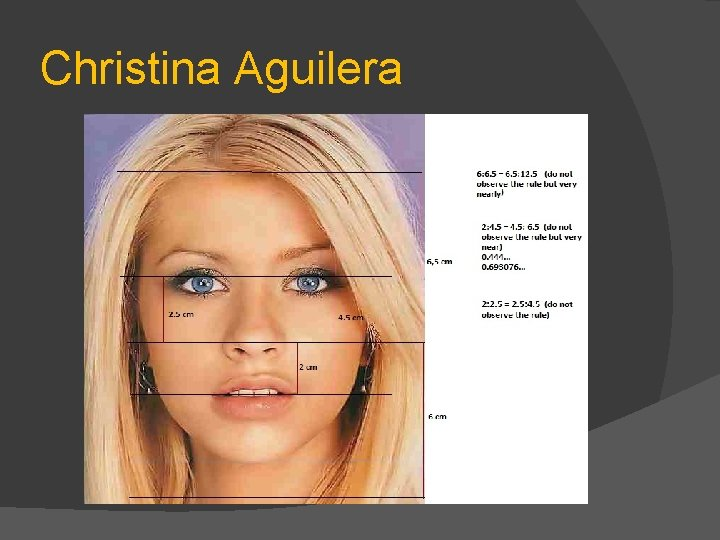Christina Aguilera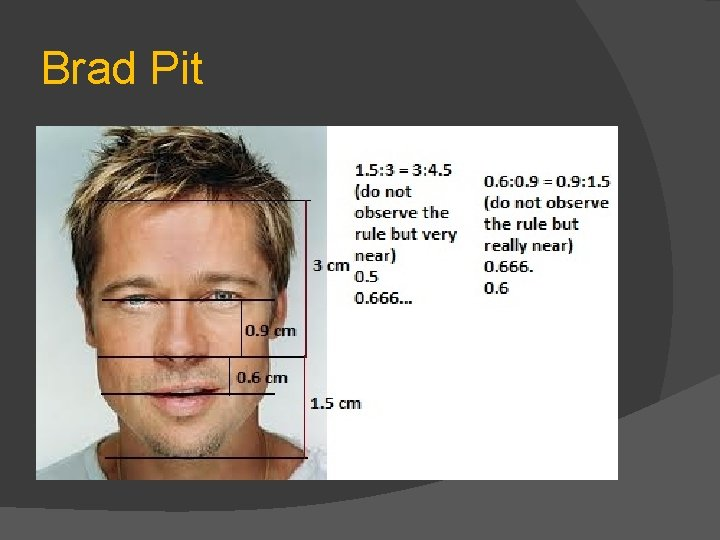Angelina JolieGOLDEN RATIO IN NATURE � Snowflake: � While the scientists were analyzing snowflake, they found Golden Ratio. Snowflake’s edges have Golden Ratio, but it is very little, so we can’t see.Golden Ratio in Virus � “Polyo Virus” consists of geometric pentagons, and these pentagons turn into another geometric figures, and scientists think of this event as an intented ratio. They found «Golden Ratio»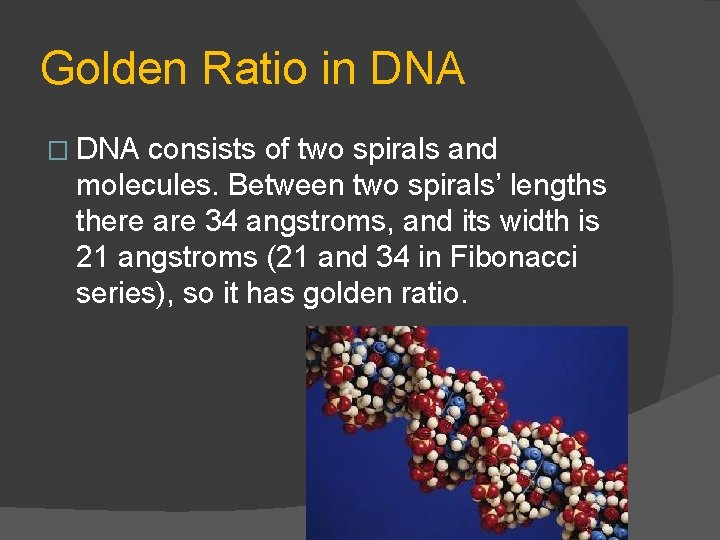Golden Ratio in DNA � DNA consists of two spirals and molecules. Between two spirals’ lengths there are 34 angstroms, and its width is 21 angstroms (21 and 34 in Fibonacci series), so it has golden ratio.� Thank you for listening Selim Furkan Tekin evrenblim. com vikipedia. org populerbilgi. com